# EV design – battery simulation

In every engineering domain using mathematical modeling and simulations brings several benefits during the design process of a system or component. In the case of battery electric vehicles, having a battery and vehicle model allows the design engineer to make prediction regarding the performance of the vehicle, function of different parameters of the high voltage battery.

There are several methods used for mathematical modeling. For a better understanding of the methods, advantages and disadvantages, read the article Methods of mathematical modeling. In our case, we are going to focus on white-box modeling, using physical principles (equations).

Since a battery is an electrochemical component/system, there are different areas which can be mathematical modelled: chemical, electrical, mechanical and thermal. In our case we are going to focus on the electrical model of the battery.

The simplest equivalent electrical circuit of battery contains a voltage source E [V] and an internal resistance Ri [Ω]. The voltage drop at the terminals of the battery U [V] depends on the value of the internal resistance and the current I [A] drawn from the battery, which depends on the resistance value of the external load (e.g. inverter, DCDC converter, etc.).

The value of the voltage drop U [V] is given by the equation:

$U = E – I \cdot R \tag{1}$

If there is no load connected to the battery, the current through the battery will be zero and the terminal voltage will be equal with the source voltage. This is called open circuit voltage.

$U = E \tag{2}$

The higher the internal resistance of the battery, the higher the voltage drop on it, the higher the power losses of the battery. For any applications, including battery electric vehicles, the value of the internal resistance should be as small as possible.

### Battery depth of discharge

The depth of discharge (DOD) of a battery is regarded as the percentage of battery capacity that has been discharged compared with the maximum capacity of the battery. The depth of discharge is the inverse of the state of charge (SOC). A battery with 100% SOC will have 0% DOD.

The open circuit voltage of a battery depends on the depth of discharge and can be approximate by empirical equations . For lead-acid batteries, the open circuit voltage is directly proportional with the state of charge of the battery and can be calculated with the following equation:

$E = n \cdot \left ( 2.15 – DOD \cdot \left ( 2.15 – 2.00 \right ) \right ) \tag{3}$

where:
n [-] – number of battery cells
DOD [-] – depth of discharge
E [V] – open circuit voltage

For nickel-cadmium batteries, the open circuit voltage is non linear and needs to be approximated by a polynomial. A good fit, produced from experimental result, is the equation :

$\begin{split} E = n \cdot \left ( -8.2816 \cdot DOD^7+23.5749 \cdot DOD^6-30 \cdot DOD^5+23.7053 \cdot DOD^4.. \\ -12.5877\cdot DOD^3+4.1315\cdot DOD^2-0.8658\cdot DOD+1.37 \right ) \quad (4) \end{split}$

We can use Scilab in order to plot the open circuit voltage for a lead-acid and a nickel-cadmium battery. In this case we are going to create a Scilab function (*.sci) which has as arguments (inputs): the number of cells, the depth of discharge and the type of battery and outputs: the open circuit voltage.

// x-engineer.org
// Function for open circuit voltage calculation
// inputs: DoD [-] - depth of discharge
//         Nc [-] - number of battery cells
//         batt_type [string] - type of battery
// output: E [V] - battery open circuit voltage
function y = Ebatt(DoD,Nc,batt_type)
y=(2.15-(2.15-2.00)*DoD)*Nc;
y=(-8.2816*DoD.^7+23.5749*DoD.^6-30*DoD.^5+23.7053*DoD.^4- ..
12.5877*DoD.^3+4.1315*DoD.^2-0.8658.*DoD+1.37)*Nc;
else
end
endfunction


The open circuit voltage function is called in another Scilab script (*.sce) which also generates the plot of the results.

// x-engineer.org
exec("Ebatt.sci") // load battery voltage function
DoD = [0:0.001:0.99]; // depth of discharge
Nc = 6; // number of cells
figure(0)
hf=gcf();
hf.background=8;
xgrid()
xlabel("Depth of discharge [%]")
ylabel("Nickel-cadmium battery (6 cells) voltage [V]")
title("x-engineer.org")
xs2png(hf, 'Nickel-cadmium battery (6 cells) voltage.png')
figure(1)
hf=gcf();
hf.background=8;
xgrid()
xlabel("Depth of discharge [%]")
ylabel("Lead-acid battery (6 cells) voltage [V]")
title("x-engineer.org")
xs2png(hf, 'Lead-acid battery (6 cells) voltage.png')


The depth of discharge is set between 0 (fully charged) and 0.99 (discharged, flat).

After running the script file, two images are going to be generated and also saved in the Scilab working folder as *.png files. The script is run for two different cases: first, only for 1 cell, second, for a battery containing 6 cells. The results can be seen in the images below.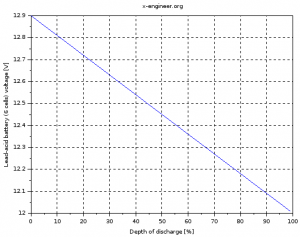Image: Lead-acid battery (6 cells) voltage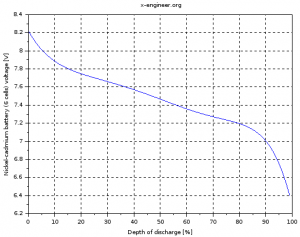Image: Nickel-cadmium battery (6 cells) voltage

The voltage of the cell (battery) is not constant but depends on the depth of discharge (state of charge) of the battery. The more discharged a battery is, the lower the voltage at the terminals.

### Battery capacity and voltage

The battery capacity, measured in Ampere-hour [Ah], gives the amount of electric charge (current) the battery can deliver during discharge, at the rated voltage. For example, a battery with the capacity of 20 Ah, can deliver 20 A for 1 h. Also, it can deliver 40 A for 0.5 h or 10 A for 2 A. As long as, the product between the electrical current [A] and discharge time [h] gives the rated capacity [Ah], the battery can theoretically deliver it.

In reality, the capacity of the battery depends on the amount of current drawn from the battery. If the current is drawn more quickly, the capacity of the battery is reduced. For example, drawing 20 A for 1 h does not take the same electrical charge as drawing 40 A for 0.5 h. The higher the current drawn from the battery, the lower the capacity. This phenomenon occurs due to the internal resistance of the battery. At higher currents, the internal resistance of the battery also increases (due to higher temperatures) which makes less voltage to be available at the battery terminals.

The battery voltage also depends on the amount of current drawn from the battery. The higher the current, the lower the voltage. This phenomenon also occurs due to the increase of the internal resistance of the battery. The higher the internal resistance, the higher the voltage drop on it, the lower the voltage at the battery terminals.

### Battery model in Xcos

According to  the state of charge of a battery can be estimated by taking into account the amount of electrical current which is going in and out of the battery.

$\text{SOC} = \text{SOC}_{0} – \int \frac{i}{Q(i)} dt \tag{5}$

where:
SOC – state of charge [-]
SOC0 – initial state of charge [-]
i – electrical current [A]
Q – battery capacity [Ah]

Based on equation (5) and using input battery parameters, we can create an Xcos block diagram model for the estimation of the battery state of charge.

The Integrator is initialised with the start value of the battery state of charge. The input signal Ibatt [A] is the current drawn from the battery, which is divided by the battery capacity. When the battery is discharged, the input Ibatt [A] is positive but it’s converted to negative for the Integrator since it needs to remove charge. When the battery is charged, the input Ibatt [A] is negative and it’s converted to positive for the Integrator since it adds charge.

The outputs of the model will be the battery state of charge SOC [%] and the battery voltage Ubatt [V]. Since the voltage depends on the battery state of charge, a liner interpolation block Interp is used to compute the battery voltage.

The parameter of the model can be defined in an Scilab script (*.sce).

bp.C_Ah = 93.6; // battery capacity
bp.SOC_x_pct = [10 12 15 20 25 85 92 95 98 100]; // battery state of charge axis
bp.U_y_V = [350 370 385 393 395 405 409 415 430 450]; // battery voltage map
bp.UDC_V = 400; // battery nominal voltage
bp.SOC0_pct = 100; // battery initial state of charge


The battery voltage is considered to be minimum 350 V at 10 % SOC (fully discharged), nominal 400 V at 55 % SOC and maximum 450 V at 100 % SOC (fully charged). The battery model can be run in a simulation where the input current (the current drawn from the battery) is simulated with a Sinus wave (Current generator). The Clock block samples the saved variable sOut at 1 s.

The electrical current has an amplitude I0 of 150 A, with a frequency ω of 0.001 rad/s. Since we want to capture an increase of the input current from 0 to 150 A and a decrease from 150 to 0 A, the simulation time tsim [s] is calculated as:

$t_{sim} = \frac{2 \cdot \pi}{\omega} \tag{6}$

The simulation parameters can also be included in the Scilab script (*.sce).

// Electrical current generator (sinus)
I0_A = 150; // current amplitude
omega_radps = 0.001; // current frequency
simTime_s = 2*%pi/omega_radps; // simulation time


The input current, battery state of charge and battery voltage are saved in a Scilab parameter sOut which can be found in the workspace at the end of the simulation. Running the simulation model and plotting the results, gives: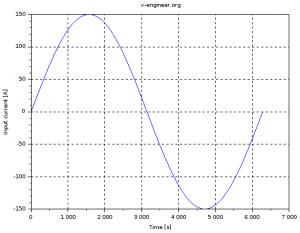Image: Battery simulation – input current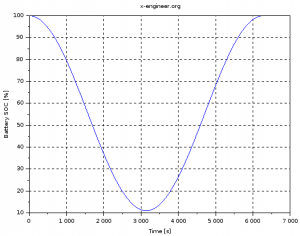Image: Battery simulation – state of charge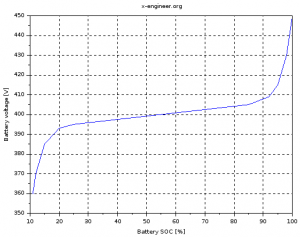Image: Battery simulation – voltage (SOC)

With the input current, the battery is discharge to 10 % state of charge in around 3000 s. Afterwards, it is increased back to 100 % SOC receiving a charging current. The battery voltage drops also to 350 V when the state of charge is at its minimum.

This model is very simple but good enough to give an estimation of the state of charge of the battery when the vehicle is driven on a standard cycle. With this model, integrated into a vehicle model, the range can be estimated for several standard or custom driving cycles.

### References:

 James Larminie, John Lowry, Electric Vehicle Technology Explained. John Wiley & Sons, 2003.
 M. Ehsani, Y. Gao, A. Emadi, Modern Electric, Hybrid Electric, and Fuel Cell Vehicles – Fundamentals, Theory, and Design, 2nd edition, CRC Press, 2010.